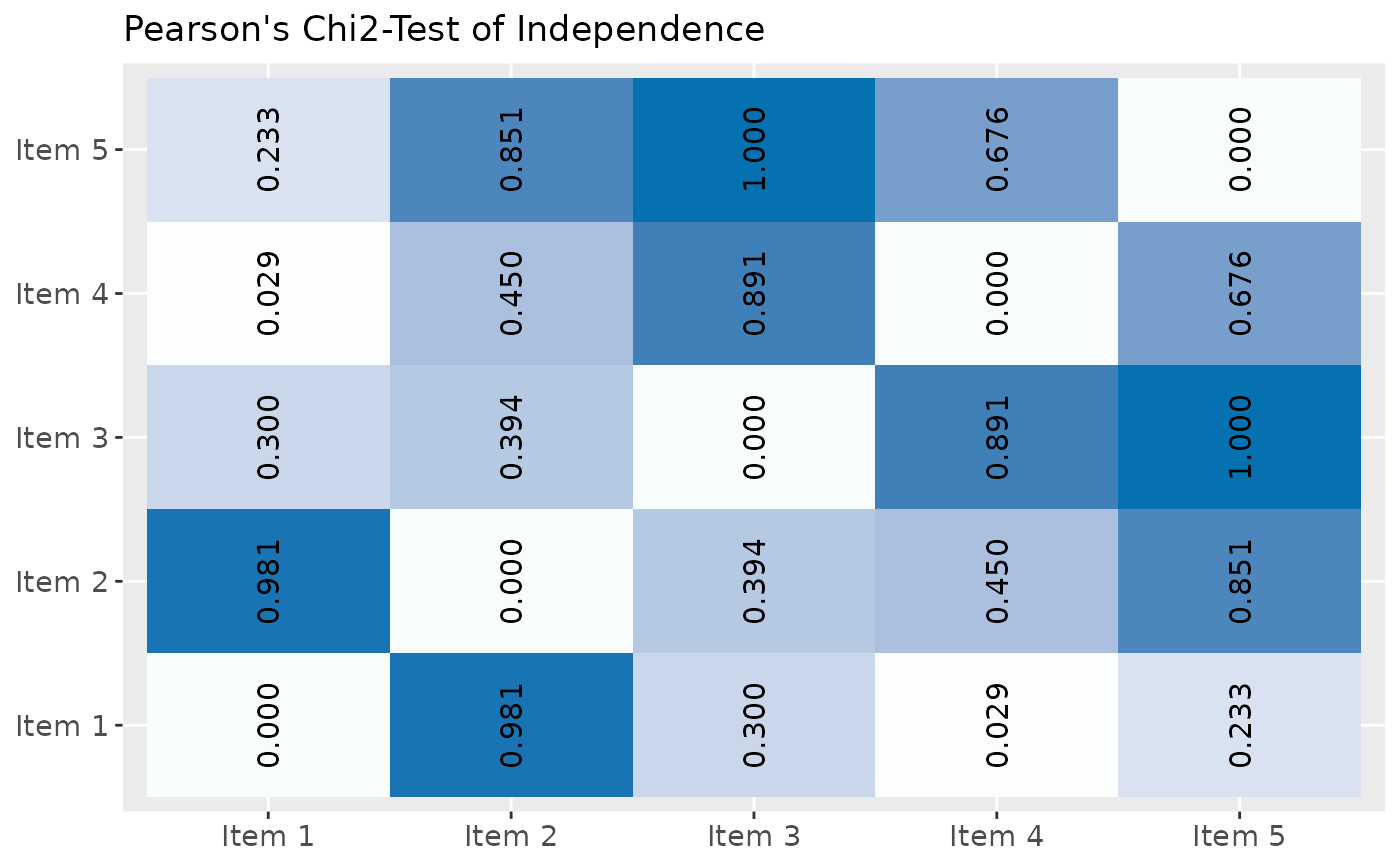Plot p-values of Pearson's Chi2-tests for multiple contingency tables as ellipses or tiles. Requires a data frame with dichotomous (dummy) variables. Calculation of Chi2-matrix taken from Tales of R.

sjp.chi2(
df,
title = "Pearson's Chi2-Test of Independence",
axis.labels = NULL,
wrap.title = 50,
wrap.labels = 20,
show.legend = FALSE,
legend.title = NULL
)

Arguments

df

A data frame with (dichotomous) factor variables.

title

character vector, used as plot title. Depending on plot type and function, will be set automatically. If title = "", no title is printed. For effect-plots, may also be a character vector of length > 1, to define titles for each sub-plot or facet.

axis.labels

character vector with labels used as axis labels. Optional argument, since in most cases, axis labels are set automatically.

wrap.title

numeric, determines how many chars of the plot title are displayed in one line and when a line break is inserted.

wrap.labels

numeric, determines how many chars of the value, variable or axis labels are displayed in one line and when a line break is inserted.

show.legend

logical, if TRUE, and depending on plot type and function, a legend is added to the plot.

legend.title

character vector, used as title for the plot legend.

A ggplot-object.

Examples

# create data frame with 5 dichotomous (dummy) variables
mydf <- data.frame(as.factor(sample(1:2, 100, replace=TRUE)),
as.factor(sample(1:2, 100, replace=TRUE)),
as.factor(sample(1:2, 100, replace=TRUE)),
as.factor(sample(1:2, 100, replace=TRUE)),
as.factor(sample(1:2, 100, replace=TRUE)))
# create variable labels
items <- list(c("Item 1", "Item 2", "Item 3", "Item 4", "Item 5"))

# plot Chi2-contingency-table
sjp.chi2(mydf, axis.labels = items)